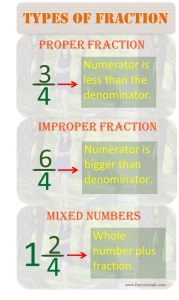>

## Types of Fraction

There are three types of fractions. Each type has its own use and has different form and properties from each other. The types of fractions are proper fraction, improper fraction, and mixed number.Proper fraction is a normal fraction as fractions are parts of a whole. When a whole is divided into parts, each part is not greater than the whole parts. The individual part is called proper fraction. Proper fraction is a fraction whose numerator is less than the denominator. This type of fraction is being used commonly and easier to deal with using different math operations. It is easier to add, subtract, multiply, and divide proper fraction.

Improper fraction is different from proper fraction. The fraction is just a part of a whole but improper fraction has different value. The value of improper fraction is greater the sum of the whole parts. Hence, improper fraction is a fraction whose numerator is greater than the denominator. There are some instances when you need to add and subtract whole numbers and fraction. When adding fraction with whole number, you need to covert it first to a type where math operation is possible. So improper fraction is used when there is a need to add, subtract, multiply, and divide fractions with whole numbers.

Mixed number is a fraction involving whole number. It means whole number plus fraction. Mixed number is used when you need to simply improper fraction. Improper fraction is hard to understand. You need it to convert to mixed number in order to be understood easily. Three over two is hard to understand than one and one half so for readability, you used mixed number if ever you have an improper fraction.

Types of fractions are easier to understand using visual aid. It creates a visual representation in your head that helps you remember how to deal with different types of fractions.

Multiplying and dividing between types is easier by converting fractions to their appropriate type. Adding and subtracting is easier too. You just have to familiarize the three types of fractions and how to convert between types.

Copyright © 2019. www.fractioncalc.com                    Privacy Policy | DMCA Policy | Terms Of Service | Contact Us